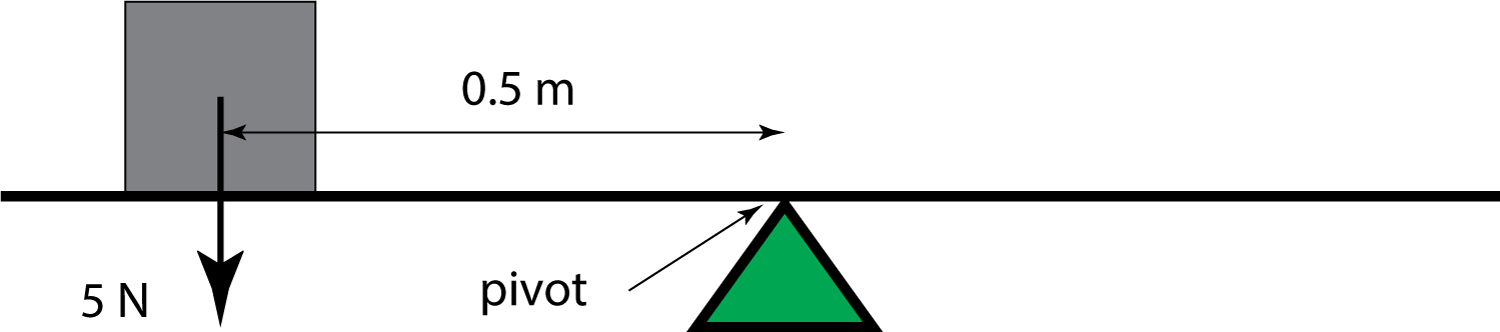µ GCSE | Moments

10 quick questions - for GCSE and iGCSE

10 minutes maximum! (can you do it in 5?)

Grab a calculator - time for some maths!

(Physics only! - Not Double Science).

1. Which one of these is the correct formula for calculating a moment?

• A. moment = force ÷ distance (at right-angles to force)
• B. moment = force x distance (at right-angles to force)
• C. moment = mass x velocity
• D. moment = mass ÷ velocity

2. A 5N box is placed on the left-hand side of a light beam as shown:What is the moment produced by the box?

• A. 10 Nm clockwise.
• B. 2.5 Nm clockwise.
• C. 10 Nm anti-clockwise.
• D. 2.5 Nm anti-clockwise.

3. A second box of weight 10 N is added to the beam. Where should it be placed to make the beam balance?

• A. 0.25 m to the right of the pivot.
• B. 1 m to the right of the pivot.
• C. 0.25 m to the left of the pivot.
• D. 1 m to the left of the pivot.

4. A door handle is 0.2 m long and needs a moment of 4 Nm to turn the handle and open the door. What is the minimum force that needs to be applied to the end of the handle to open the door?

1. 0.8 N
2. 0.05 N
3. 8 N
4. 20 N

5&6. This beam has 2 weights suspended from it. However it is not balanced.5. What is the resultant moment on he beam?

1. 2 N anticlockwise
2. 8 Ncm anticlockwise
3. 2 N clockwise
4. 8 Ncm clockwise

6. To make the beam balance, how far should the 8N weight be to the right of the pivot?

1. 6 cm
2. 9 cm
3. 10 cm
4. 12 cm

7-10. These questions are about a seesaw.

When different children sit on the see-saw they find they have to move to different points to make the see-saw balance.What are the missing values needed to make the seesaw balance?

 Left Hand Side Right Hand Side Name weight (N) distance from pivot (m) Name Weight (N) distance from pivot (m) 7. Abby 600 1.5 Charlie 900 ? 1 1.5 2 135 8. Borat 750 ? 0.83 1 1.2 1.5 Elin 450 2 9. Doris ? 798 407 1676 380 2.1 Fred 570 1.4 10. ? Abby Borat Charlie Doris Elin Fred Goh ? 1.6 Goh 400 2.4